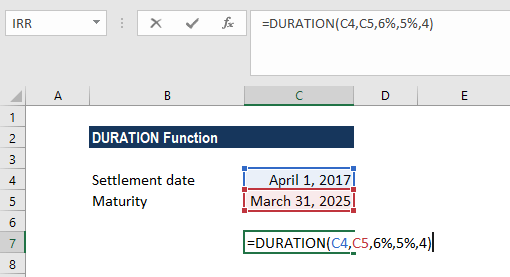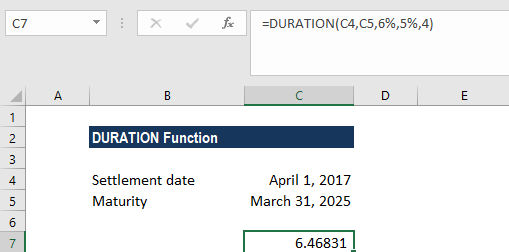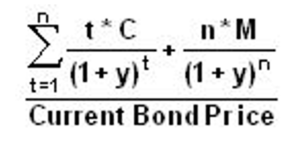# DURATION Function

Calculates the duration of a security that pays interest on a periodic basis

## What is the DURATION Function?

The DURATION function is categorized under Excel Financial functions. It helps to calculate the Macauley Duration. The function calculates the duration of a security that pays interest on a periodic basis with a par value of \$100.

DURATION is commonly used by Portfolio Managers who use the immunization strategy. Apart from it, the function is also useful in financial modeling, particularly in predicting future cash flows of investments.

### Formula

=DURATION(settlement, maturity, coupon, yield, frequency, [basis])

The DURATION function uses the following arguments:

1. Settlement (required argument) – This is the security’s settlement date or the date on which the coupon is purchased.
2. Maturity (required argument) – The security’s maturity date or the date on which the coupon expires.
3. Coupon (required argument) – This is the security’s coupon rate.
4. Yield (required argument) – The security’s annual yield.
5. Frequency (required argument) – This is the number of coupon payments per year. For annual payments, the frequency is = 1; for semiannual, frequency is = 2; and for quarterly, frequency = 4.
6. Basis (optional argument) – This is the type of day count basis to be used. The possible values of the basis are:

BasisDay Count basis
0 or omittedUS(NASD) 30/360
1Actual/actual
2Actual/360
3Actual/365
4European 30/360

Remember that the date arguments should be encoded into the DURATION function as either:

• References to cells containing dates
• Dates returned from formulas

If we try to input text representations of dates into Excel functions, they may be interpreted differently, depending on the date system and date interpretation settings on our computer.

### How to use the DURATION Function in Excel?

As a worksheet function, DURATION can be entered as part of a formula in a cell of a worksheet. To understand the uses of the function, let us consider a few examples:

#### Example 1

In this example, we will calculate the duration of a coupon purchased on April 1, 2017, with a maturity date of March 31, 2025, and a coupon rate of 6%. The yield is 5% and payments are made quarterly.The function returns a duration of 6.46831 years.As we omitted the basis argument, the DURATION function took the days count as US(NASD) 30/360. As it uses Macaulay Duration, the formula used is:### Things to remember about the DURATION Function:

1. #NUM! error – Occurs if either:
1. The supplied settlement date is ≥ maturity date; or
2. Invalid numbers are supplied for the coupon, yld, frequency, or [basis] arguments, i.e. if either: coupon < 0; yld < 0; frequency is not equal to 1, 2, or 4; or [basis] is supplied and is not equal to 0, 1, 2, 3, or 4).
2. #VALUE! error – Occurs if either:
1. Any of the given arguments is non-numeric
2. One or both of the given settlement or maturity dates are not valid Excel dates.
3. Settlement, maturity, frequency, and basis are truncated to integers.
4. In MS Excel, dates are stored as sequential serial numbers so they can be used in calculations. By default, January 1, 1900 is serial number 1, and January 18, 2018 is serial number 43118, because it is 43,118 days after January 1, 1900.

Thanks for reading CFI’s guide to important Excel functions! By taking the time to learn and master these functions, you’ll significantly improve your financial modeling. To learn more, check out these additional CFI resources:

• Excel for Finance